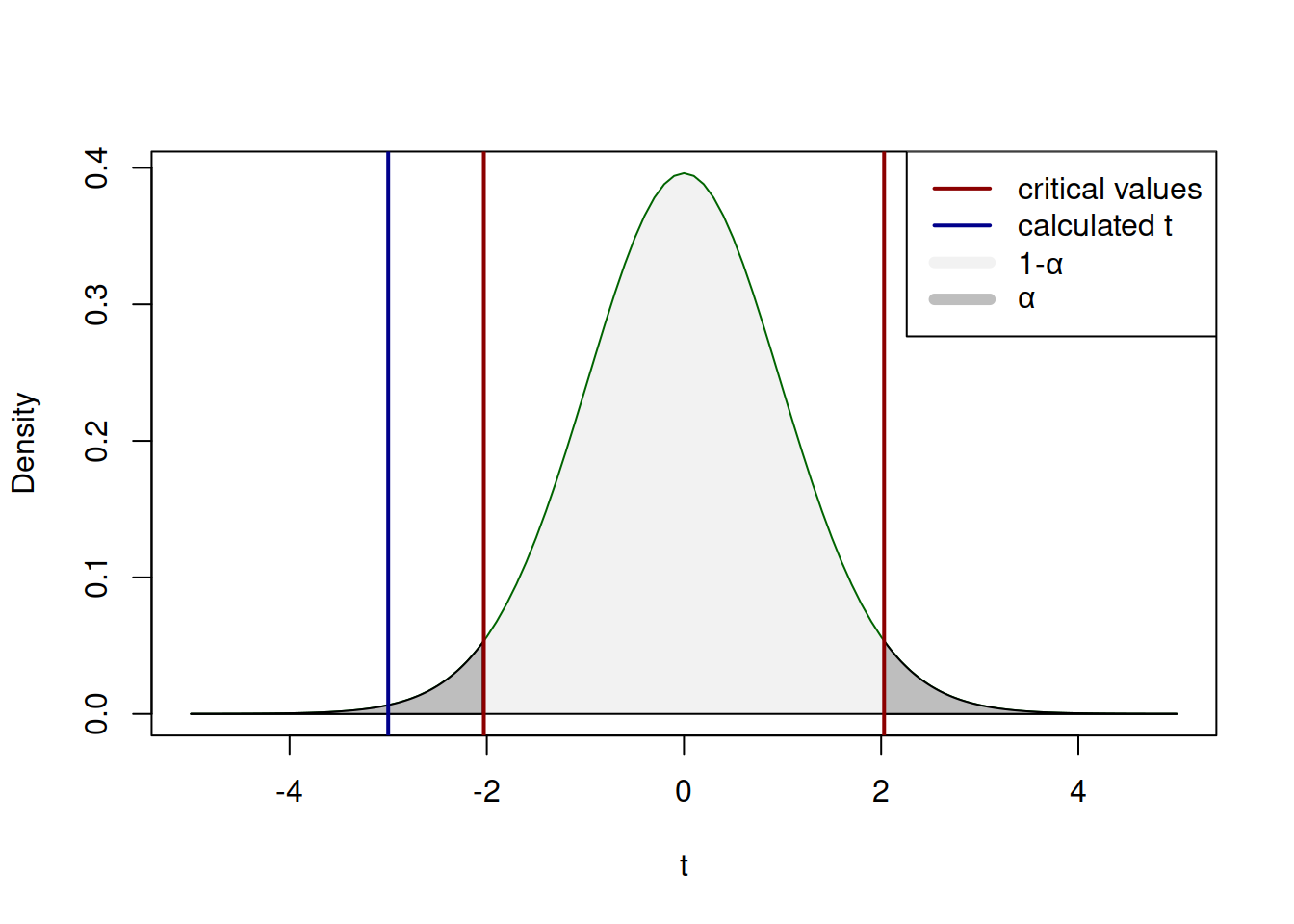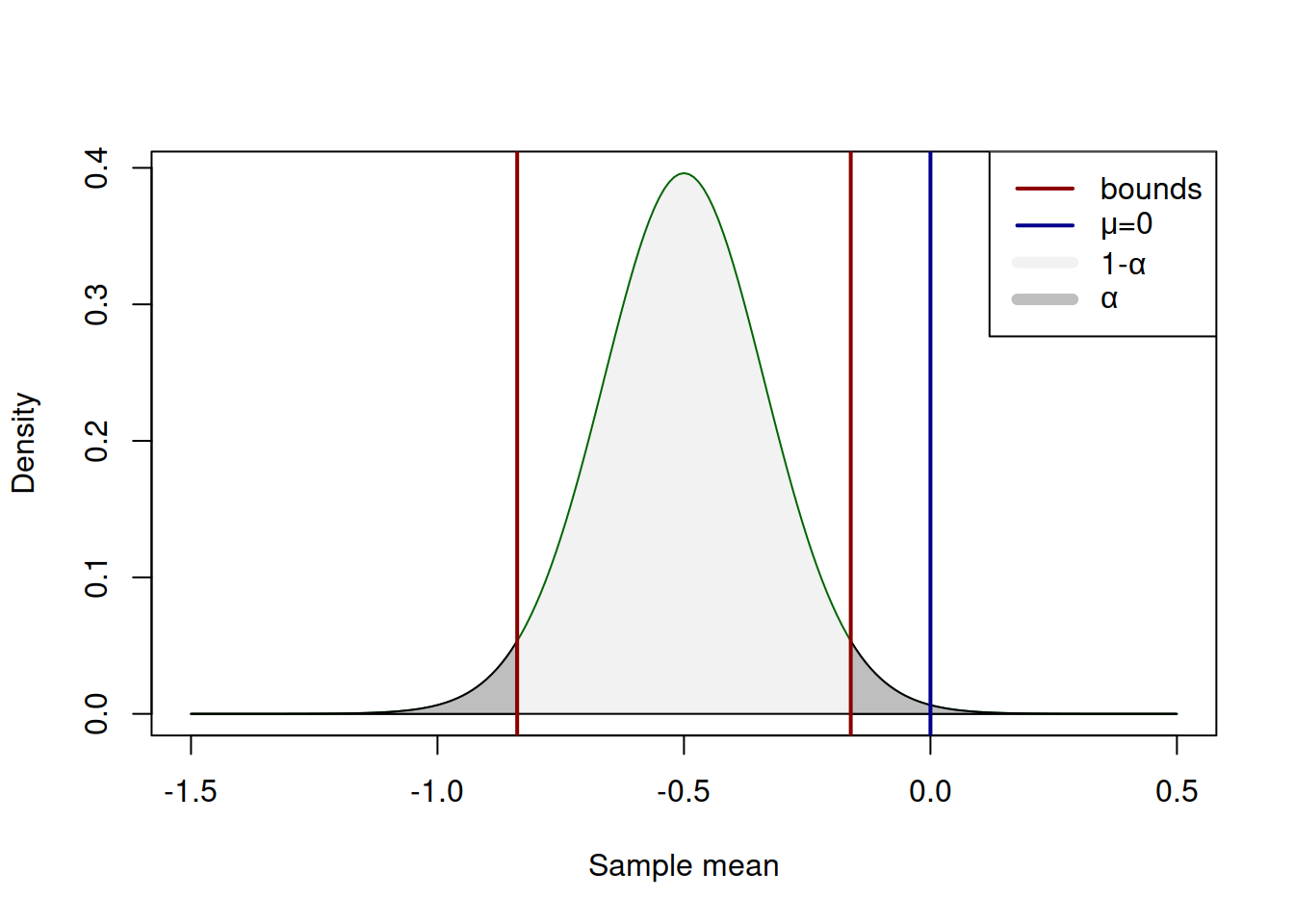This book is in Open Review. I want your feedback to make the book better for you and other readers. To add your annotation, select some text and then click the on the pop-up menu. To see the annotations of others, click the button in the upper right hand corner of the page

## 2.3 Hypothesis testing

While we will not need hypothesis testing for ADAM itself, we might face it in some parts of the textbook, so it is worth discussing it briefly.

Hypothesis testing arises naturally from the idea of confidence intervals: instead of constructing the interval and getting the idea about the uncertainty about the parameter, we could check, whether the sample agrees with our expectations or not. For example, we could test, whether the population mean is equal to zero based on our sample. We could either construct a confidence interval for the sample mean and see if zero is included in it (in which case it might indicate that zero is one of the possible values of the population mean), or we could reformulate the problem and compare some calculated value with the theoretical threshold one. The latter approach is in the nutshell what hypothesis testing does.

Fundamentally, the hypothesis testing process relies on the ideas of induction and dichotomy: we have a null (H$$_0$$) and alternative (H$$_1$$) hypotheses about the process or a property in the population, and we want to find some evidence to reject the H$$_0$$. Rejecting a hypothesis is actually more useful than not rejecting it, because in the former case we know what not to expect from the data, while in the latter we just might not have enough evidence to make any solid conclusion. For example, we could formulate H$$_0$$ that all cats are white. Failing to reject this hypothesis based on the data that we have (e.g. a dataset of white cats) does not mean that they are all (in the universe) indeed white, it just means that we have not observed the non-white ones. If we collect enough evidence to reject H$$_0$$ (i.e. encountered a black cat), then we can conclude that not all cats are white. This is a more solid conclusion than the in the previous case. So, if you are interested in a specific outcome, then it makes sense to put this in the alternative hypothesis and see if the data allows to reject the null. For example, if we want to see if the average salary of professors in the UK is higher than £100k per year we would formulate the hypotheses in the following way: $\begin{equation*} \mathrm{H}_0: \mu \leq 100, \mathrm{H}_1: \mu > 100. \end{equation*}$

Having formulated hypotheses, we can check them, but in order to do that, we need to follow a proper procedure, which can be summarised in the following six steps:

1. Formulate null and alternative hypotheses (H$$_0$$ and H$$_1$$) based on your understanding of the problem;
2. Select the significance level $$\alpha$$ on which the hypothesis will be tested;
3. Select the test appropriate for the formulate hypotheses (1);
4. Conduct the test (3) and get the calculated value;
5. Compare the value in (4) with the threshold one;
6. Make a conclusion based on (5) on the selected level (2).

Note that the order of some elements might change depending on the circumstances, but (2) should always happen before (4), otherwise we might be dealing with so called "p-hacking", trying to make results look nicer than they really are.

Consider an example, where we want to check, whether the population mean $$\mu$$ is equal to zero or not based on a sample of 36 observations, where $$\bar{y}=-0.5$$ and $$s^2=1$$. In this case, we formulate the null and alternative hypotheses: $\begin{equation*} \mathrm{H}_0: \mu=0, \mathrm{H}_1: \mu \neq 0. \end{equation*}$ We then select the significance level $$\alpha=0.05$$ (just as an example) and select the test. Based on the description of the task, this can be either a t-test, or a z-test, depending on whether the variance of the variable is known or not. Usually it is not, so we tend to use t-test. We then conduct the test using the formula: $\begin{equation} t = \frac{\bar{y} - \mu}{s_{\bar{y}}} = \frac{-0.5 - 0}{\frac{1}{\sqrt{36}}} = -3 . \tag{2.6} \end{equation}$

After that we get the critical value of t with $$df=36-1=35$$ degrees of freedom and significance level $$\alpha/2=0.025$$, which is approximately equal to -2.03. We compare this value with the (2.6) by modules and reject H$$_0$$ if the calculated value is higher than the critical one. In our case it is, so it appears that we have enough evidence to say that the population mean is not equal to 0, on the 5% significance level.

Visually, the whole process of hypothesis testing explained above can be represented in the following way:Figure 2.6: The process of hypothesis testing with t value

If the blue line on Figure 2.6 would lie inside the red bounds (i.e. the calculated value is less than the critical value by module), then we would fail to reject H$$_0$$. But in our example it is outside the bounds, so the we have enough evidence to conclude that the population mean is not equal to zero on 5% significance level. Notice, how similar the mechanisms of confidence interval construction and hypothesis testing are. This is because they are one and the same thing, presented differently. In fact, we could test the same hypothesis by constructing the 95% confidence interval using (2.4) and checking, whether the interval covers the $$\mu=0$$: \begin{equation*} \begin{aligned} & \mu \in \left(-0.50 -2.03 \frac{1}{\sqrt{36}}, -0.50 + 2.03 \frac{1}{\sqrt{36}} \right), \\ & \mu \in (-0.84, -0.16). \end{aligned} \end{equation*} In our case it does not, so we conclude that we reject H$$_0$$ on 5% significance level. This can be roughly represented by the graph on Figure 2.7:Figure 2.7: The process of hypothesis testing with t value

Note that the positioning of the blue line has changed in the case of confidence interval, which happens because of the transition from (2.6) to (2.4). The idea and the message, however, stay the same: if the value is not inside the light grey area, then we reject H$$_0$$ on the selected significance level.

Finally, there is a third way to test the hypothesis. We could calculate how much surface is left in the tails with the cut off of the assumed distribution by the blue line on 2.6 (calculated value). In R this can be done using the pt() function:

pt(-3, 36-1)
##  0.002474416

Given that we had the inequality in the alternative hypothesis, we need to consider both tails, multiplying the value by 2 to get approximately 0.0049. This is the significance level, for which the switch from "reject" to "do not reject" happens. We could compare this value with the pre-selected significance level directly, rejecting H$$_0$$ if it is lower than $$\alpha$$. This value is called "p-value" and simplifies the hypothesis testing, because we do not need to look at critical values or construct the confidence interval. There are different definitions of what it is, I personally find the following easier to comprehend: p-value is the smallest significance level at which a null hypothesis can be rejected.

Despite this simplification, we still need to follow the procedure and select $$\alpha$$ before conducting the test! We should not change the significance level after observing the p-values, otherwise we might end up bending reality for out needs.

Also note that we never say that we accept H$$_0$$, because this is not what we do in hypothesis testing: if the value would lie inside the interval, then this would only mean that our sample shows that the tested value is covered by the region - the true value can be any of the numbers between the bounds.

While p-value is a comfortable instrument, I personally prefer using confidence interval, because they show the uncertainty clearer. Consider the following cases to see what I mean:

1. We reject H$$_0$$ because t-value is -3, which is smaller than the critical value of -2.03 (or equivalently the absolute of t-value is 3, while the critical is 2.03);
2. We reject H$$_0$$ because p-value is 0.0049, which is smaller than the significance level $$\alpha=0.05$$;
3. The confidence interval for the mean is $$\mu \in (-0.84, -0.16)$$. It does not include zero, so we reject H$$_0$$.

In case of (3), we not only get the same message as in (1) and (2), but we also see how far the bound is from the tested value. In addition, in the situation, when we fail to reject H$$_0$$, the approach (3) gives more appropriate information. Consider the case, when we test, whether $$\mu=-0.6$$. We then have the following three approaches to the problem:

1. We fail to reject H$$_0$$ because t-value is 0.245, which is smaller than the critical value of 2.03;
2. We fail to reject H$$_0$$ because p-value is 0.808, which is greater than the significance level $$\alpha=0.05$$;
3. The confidence interval for the mean is $$\mu \in (-0.84, -0.16)$$. It includes -0.6, so we fail to reject H$$_0$$. This does not mean that the true mean is indeed equal to -0.6, but it means that the region will cover it in 95% of cases if we do resampling many times.

In my opinion, the third approach is more informative and saves from making wrong conclusions about the tested hypothesis, making you work a bit more (you cannot change the confidence level on the fly, you would need to reconstruct the interval). Having said that, either of the three is fine, as long as you understand what they really imply.

Finally, if you do hypothesis testing and use p-values, it is worth mentioning the statement of American Statistical Association about p-values (Wasserstein and Lazar 2016). Among the different aspects discussed in this statement, there is a list of principles related to p-values, which I cite below:

1. P-values can indicate how incompatible the data are with a specified statistical model;
2. P-values do not measure:
• the probability that the studied hypothesis is true,
• or the probability that the data were produced by random chance alone;
1. Scientific conclusions and business or policy decisions should not be based only on whether a p-value passes a specific threshold;
2. Proper inference requires full reporting and transparency;
3. A p-value, or statistical significance, does not measure:
• the size of an effect
• or the importance of a result;
1. By itself, a p-value does not provide a good measure of evidence regarding a model or hypothesis.

The statement provides more details about that, but summarising, whatever hypothesis you test and however you test it, you should have apriori understanding of the problem. Diving in the data and trying to see what floats (i.e. which of the p-values is higher than $$\alpha$$) is not a good idea (Wasserstein and Lazar 2016). Follow the proper procedure if you want to test the hypothesis.

### References

Wasserstein, Ronald L., and Nicole A. Lazar. 2016. “The ASA’s Statement on p-Values: Context, Process, and Purpose.” American Statistician 70 (2). Taylor & Francis: 129–33. doi:10.1080/00031305.2016.1154108.